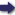(London :  Kegan Paul, Trench, Trübner & Co.,  1910.)

 Tools

## Search this bookPrev Page 75 Next``` CHAPTER LV. 75 the measure of the body would not vary (v. p. 74). Therefore the commentator Balabhadra holds the same opinion as Pulisa, viz. that the divisor in this division should be the true distance reduced (to the measure of yojctnas). Brahmagupta gives the following rule for the com- prahma- putation of the diameter of the shadow, which in our SSifodfor canones is called the measure of the sphere of the dragon's tation^f^the head and tail: " Subtract the yojanas of the diameter the^Lrdow. of the earth, i.e. 1581, from the yojctncts of the diameter of the sun, i.e. 6522. There remains 4941, which is kept in memory to be used as divisor. It is represented in the figure by AE. Further multiply the diameter of the earth, which is the double sinus totus, by the yojancts of the true distance of the sun, which is found by the correction of the sun. Divide the product by the divisor kept in memory. The quotient is the true distance of the shadow's end. "Evidently the two triangles AEC and CDH are similar to each other. However, the normal line OT does not vary in size, whilst in consequence of the true distance the ap)pectrctnce of AB varies, though its size is constantly the same. Now let this distance be CK. Draw the lines AJ and EV parallel to each other, and JKV parallel to AB. Then the latter is equal to the divisor kept in memory. " Draw the line JCM. Then M is the head of the cone of the shadow for that time. The relation of JV, the divisor kept in memory, to KC, the true distance, is the same as that of CD, the diameter of the earth, to ML, which he (Brahmagupta) calls a true distance (of Page 240. the shadow's end), and it is determined by the minutes of the sine (the earth's radius being the sinus totus). For KC-------" Now, however, I suspect that in the following some- Lacuna in ' ' -•■ _ '-' the manu- thina: has fallen out in the manuscript, for the author script copy o ■•• . of Brahma- continues : " Then multiply it (i.e. the quotient of CK, gupta. ```Prev Page 75 Next